## Scatter Plot in R¶

In this post, we will learn how make scatter plots using R and the package ggplot2. This is the notebook for the scatter plot in R tutorial: https://www.marsja.se/how-to-make-a-scatter-plot-in-r-with-ggplot2/

## Required r-packages¶

You need to install the packages used in this tutorial packages before continuing.

### How to Install R-packages¶

You install packages with the install.packages() function. Make sure to uncomment (remove the '#') if you actually need to install the packages!

In :
# install.packages(c("tidyverse", "GGally"))


Here's the single packages used in the tutorial, if you only want those installed;

In :
# to.install <- c("magittr", "purrr",
#  "ggplot2", "dplyr", "broom", "GGally")
# install.packages(to.install)


## How to Make a Scatter Plot in R¶

Time to learn how to produce a scatter plot using R statistical programming environment and we start by using the mtcars dataset.

In :
require(ggplot2)


Loading required package: ggplot2
Warning message:
"package 'ggplot2' was built under R version 3.6.1"
mpgcyldisphpdratwtqsecvsamgearcarb
Mazda RX421.0 6 160 110 3.90 2.62016.460 1 4 4
Mazda RX4 Wag21.0 6 160 110 3.90 2.87517.020 1 4 4
Datsun 71022.8 4 108 93 3.85 2.32018.611 1 4 1
Hornet 4 Drive21.4 6 258 110 3.08 3.21519.441 0 3 1
Hornet Sportabout18.7 8 360 175 3.15 3.44017.020 0 3 2
Valiant18.1 6 225 105 2.76 3.46020.221 0 3 1

Data can, also, be stored in Excel files:

## How to use Ggplot2 to Produce Scatter Plots in R¶

In this section we will learn how to make scattergraphs in R using ggplot2.

### How to Make a Scatter Plot in R¶

We will start by visualizing the variables wt (x-axis) and mpg (y-axis).

Before going on and creating the first scatter plot in R we will briefly cover ggplot2 and the plot functions we are going to use. First, we start by using ggplot to create a plot object.

Inside of the ggplot() function, we’re calling the aes() function that describe how variables in our data are mapped to visual properties . In this simple scatter plot in R example, we only use the x- and y-axis arguments and ggplot2 to put our variable wt on the x-axis, and put mpg on the y-axis.

In :
require(ggplot2)

gp <- ggplot(aes(x = wt, y = mpg),
data = mtcars)

gp + geom_point()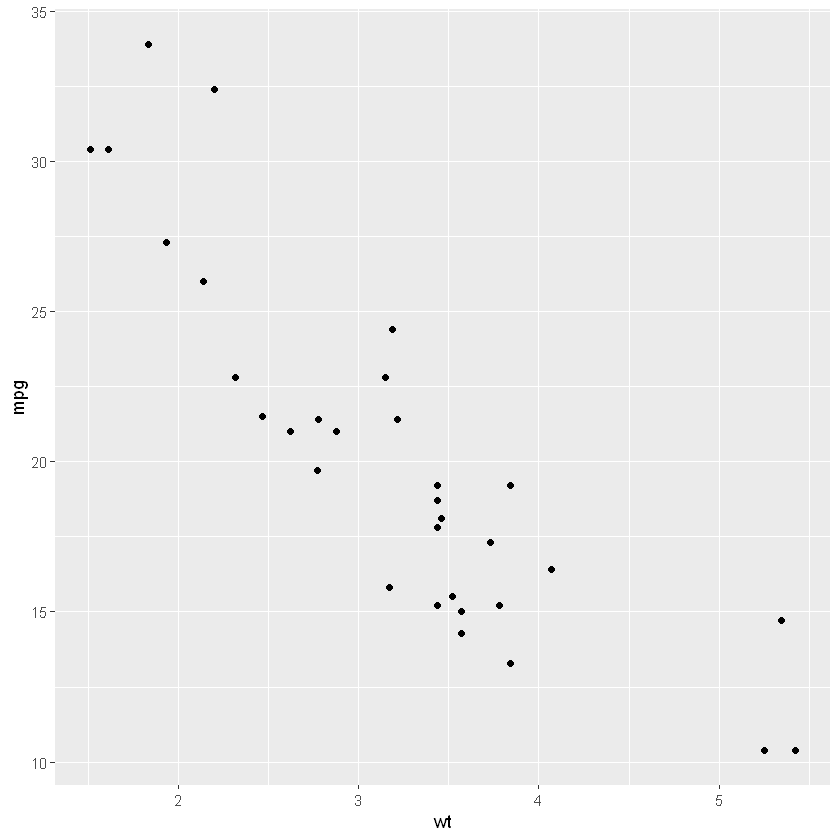### How to Change the Size of the Dots in a Scatter Plot¶

Here we'' change the size of the markers size using the size argument.

In :
gp + geom_point(size = 4)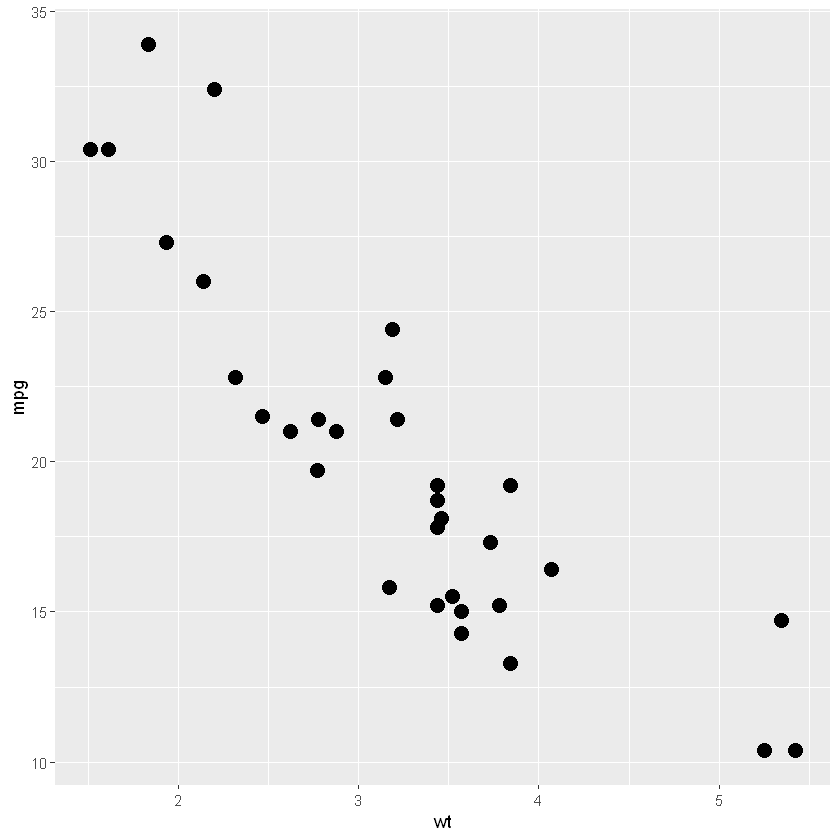Note, we used aes() but added the size argument to the geom_point() function.

In :
gp + geom_point(aes(size = wt))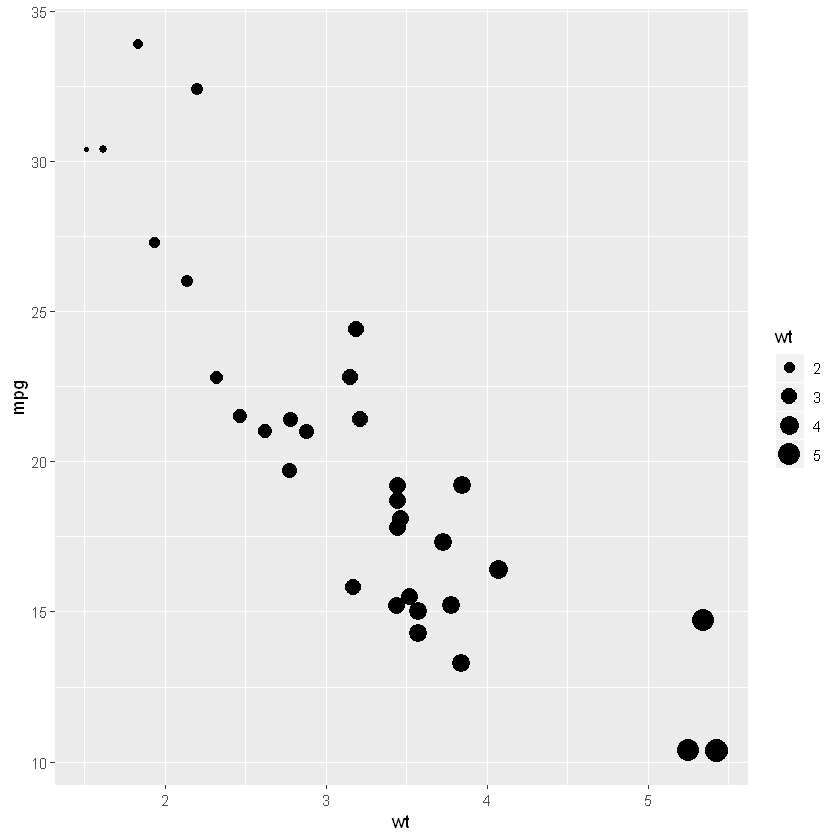#### How to Change the Number of ´ticks using ggplot2¶

To change the x-axis we use the function scale_x_continuous and to change the y-axis we use the function scale_y_continuous. Furthermore, we use the arguments limits, which take a vector, and we can set the limits to change the ticks.

In :
gp <- ggplot(aes(x = wt, y = mpg),
data = mtcars) +
geom_point()

gp + scale_y_continuous(limits=c(1, 40)) +
scale_x_continuous(limits=c(0, 6))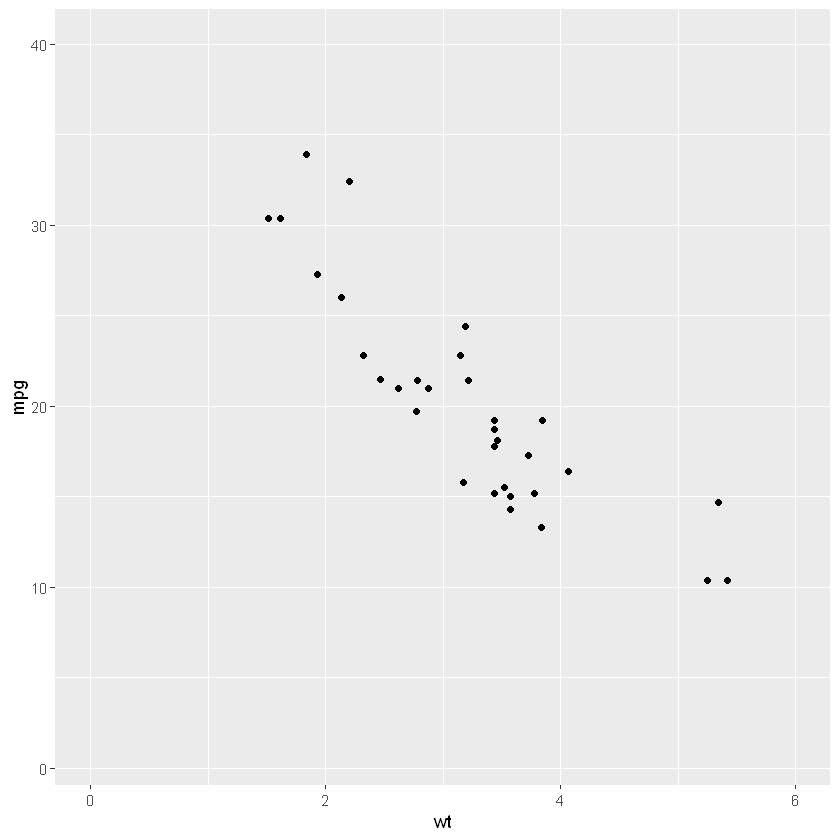Next we also change the number of ticks by adding the breaks argument to the above functions. Furthermore, we add the seq function to create a numeric vector.

In :
gp + scale_y_continuous(limits=c(1, 35),
breaks=seq(1, 35, 5)) +
scale_x_continuous(limits=c(1.5, 5.5),
breaks=seq(1.5, 5.5, 1))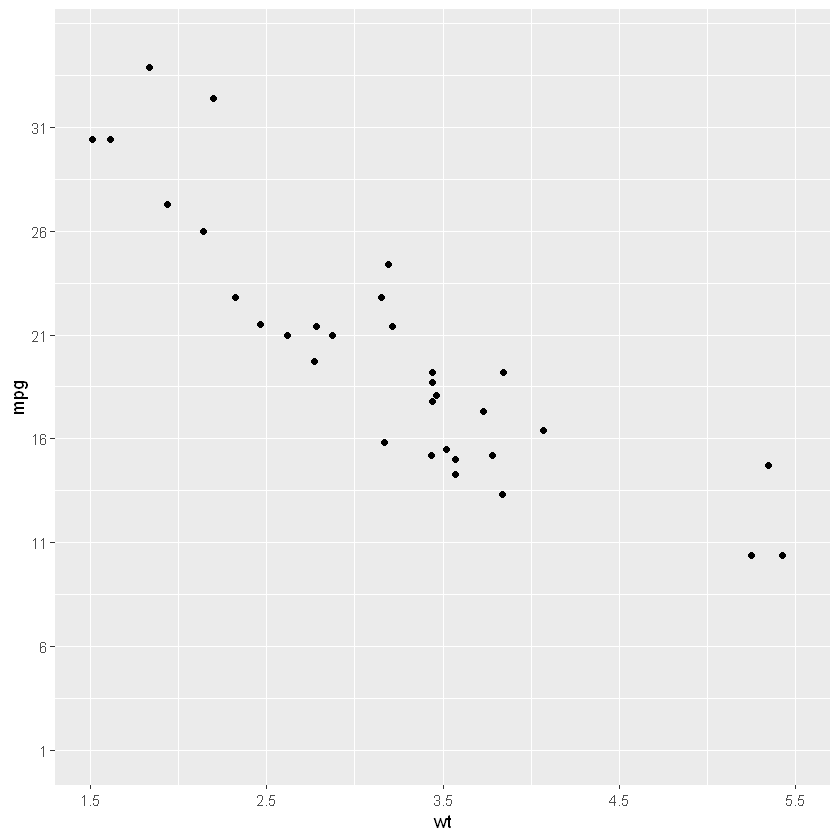#### Grouped Scatter Plot in R¶

Here we group by using color argument and the factor function to change the variable vs to a factor.

In :
gp <- ggplot(aes(x=wt, y=mpg, color=factor(vs)),
data=mtcars)
gp + geom_point()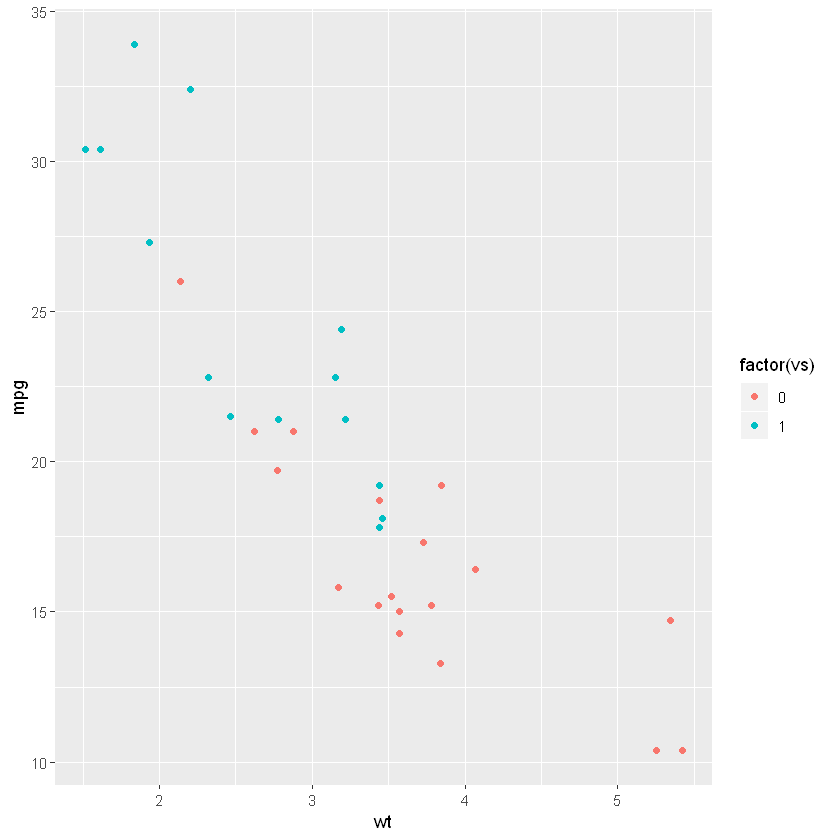Another option is using the as.factor function and change vs to a factor in the dataframe object.

In :
mtcars$vs <- as.factor(mtcars$vs)
gp <-ggplot(aes(x=wt, y=mpg, color=vs),
data=mtcars)
gp + geom_point()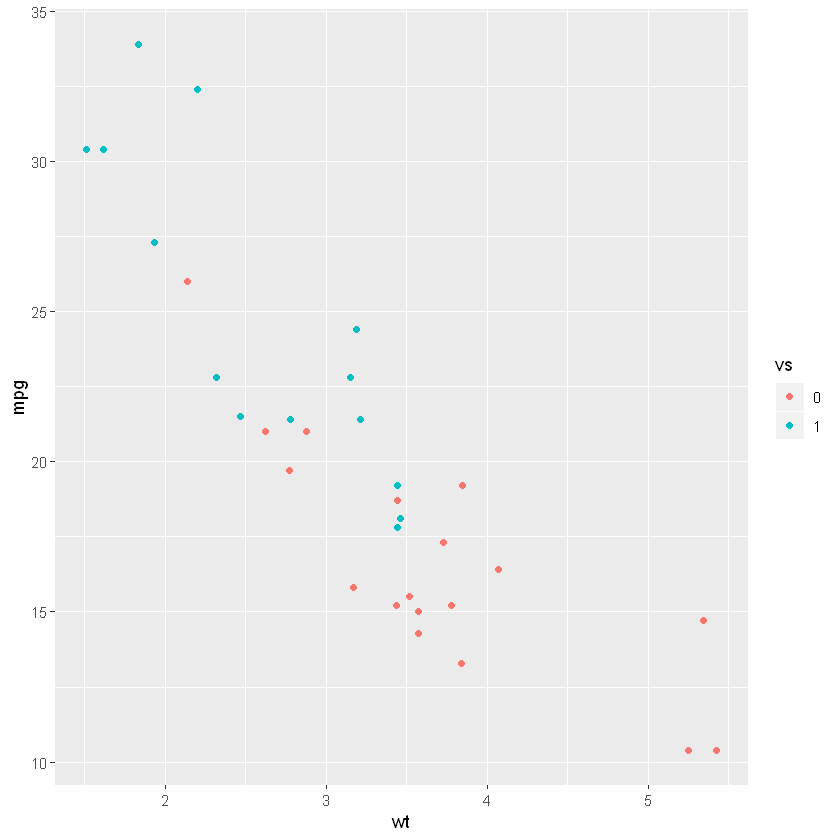#### Changing the Markers (the dots)¶

Here we are adding thea aes() function in the geom_point() function. In the aes() function we are adding the color and shape arguments and add the class column (the categorical variable).

In :
data(Burt, package = 'carData')
Burt$class <- as.factor(Burt$class)

gp <- ggplot(aes(x = IQbio, y = IQfoster), data = Burt)
gp + geom_point(aes(color = class,
shape = class))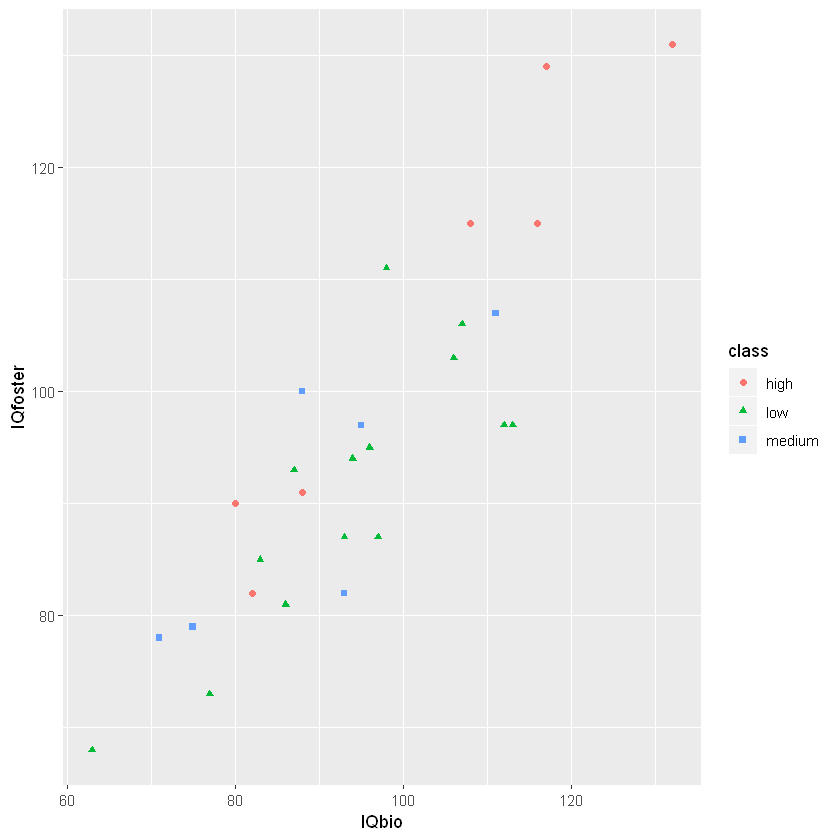### How to Add a Trend Line to a Scatter Plot in R¶

We use the geom_smooth() function and the method “lm” to add a regression line.

In :
gp <- ggplot(aes(x = IQbio, y = IQfoster), data = Burt)
gp + geom_point(aes(color = class,
shape = class)) +
geom_smooth(method = "lm", se = FALSE)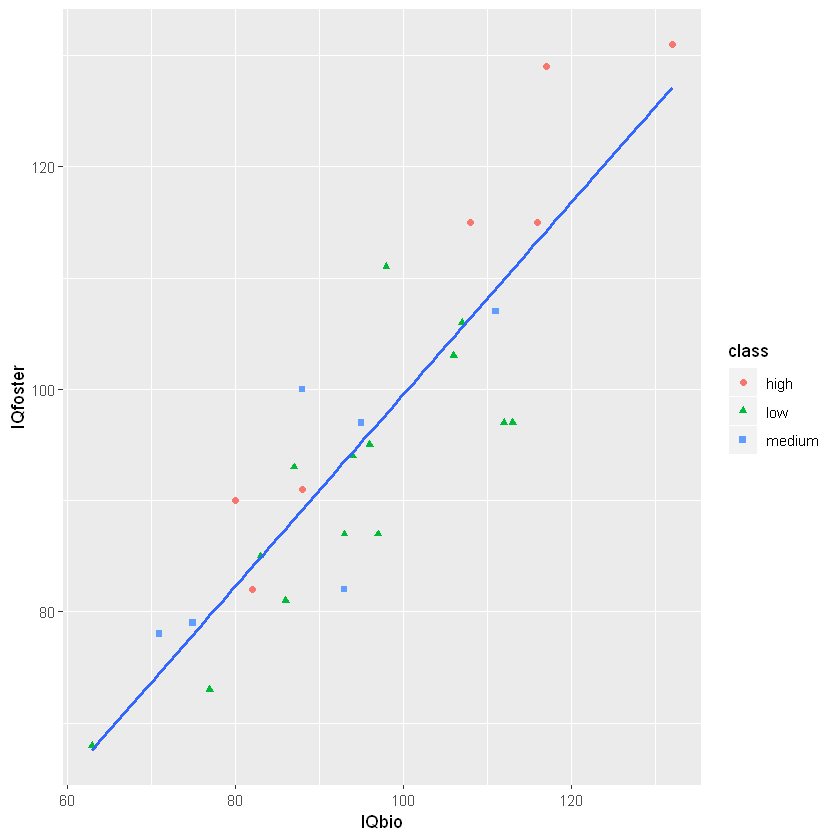In the next scatter plot example, we are going to add a regression line to the plot for each factor (category) also. Remember, we just add the color and shape arguments to the geom_point() function:

In :
gp + geom_point(aes(color = class,
shape = class)) +
geom_smooth(aes(color = class), method = "lm", se = FALSE)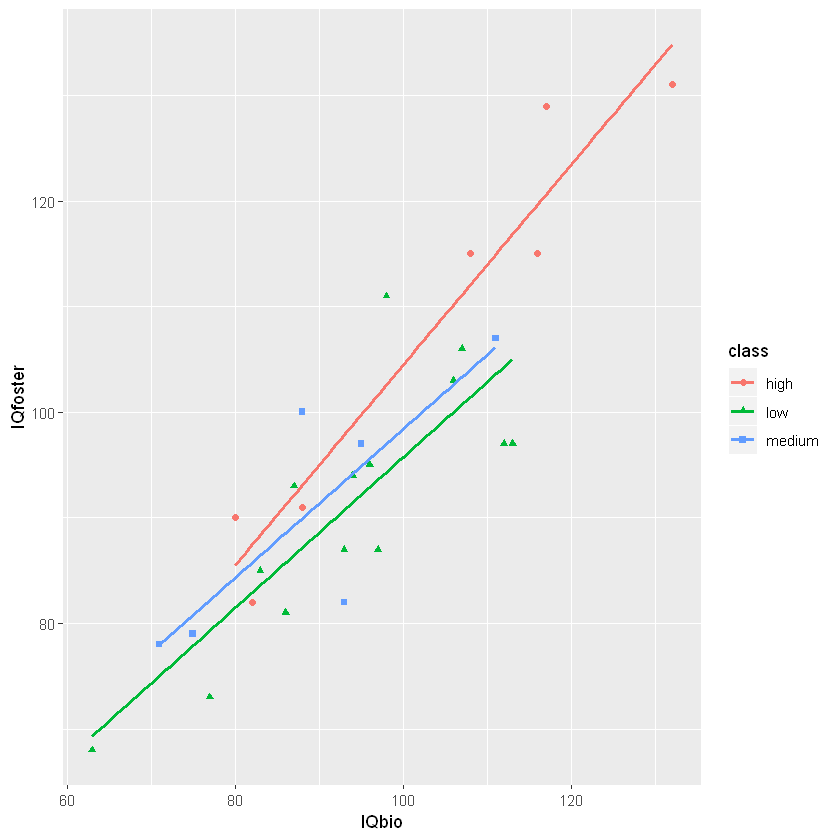#### Bivariate Distribution on a Scatter plot¶

We are adding a bivariate distribution on the scatter plot in R using the geom_density2d() function.

In :
gp <- ggplot(aes(x=wt, y=mpg),
data=mtcars)
gp + geom_point() + geom_density2d()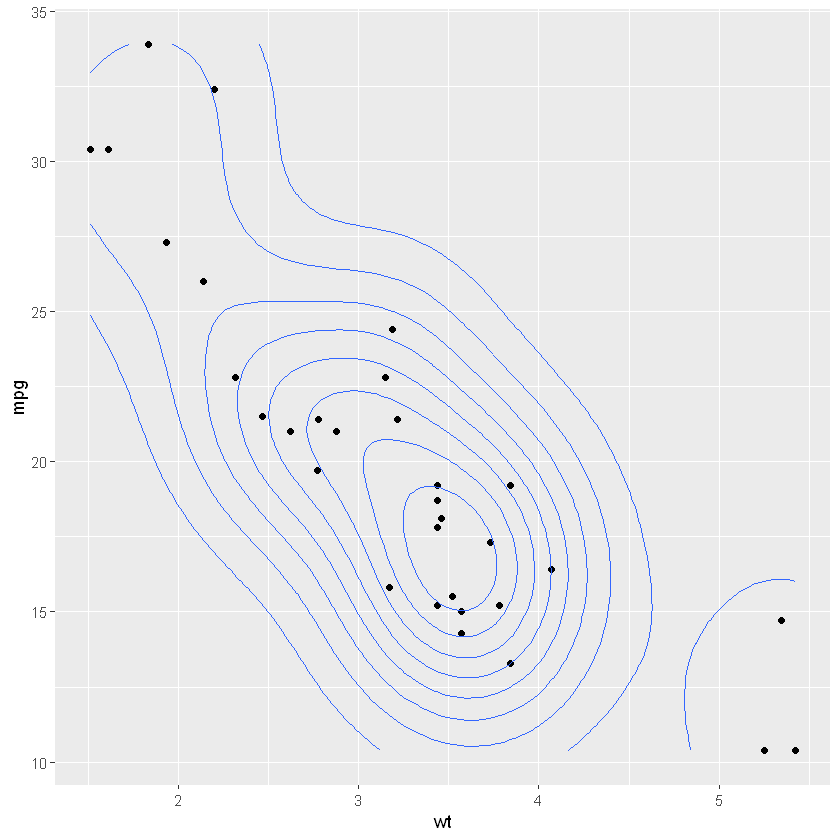### How to Add Text to Scatter Plot in R¶

Let's carry out correlation analysis using R, extract the r– and p-values, and later learn how to add this as text to our scatter plot.

In :
require(dplyr)
require(broom)

corr <- mtcars  %$% cor.test(mpg, wt) %>% tidy %>% mutate_if(is.numeric, round, 4) corr  Loading required package: dplyr Attaching package: 'dplyr' The following objects are masked from 'package:stats': filter, lag The following objects are masked from 'package:base': intersect, setdiff, setequal, union Loading required package: broom Warning message: "package 'broom' was built under R version 3.6.1" estimatestatisticp.valueparameterconf.lowconf.highmethodalternative -0.8677 -9.559 0 30 -0.9338 -0.7441 Pearson's product-moment correlationtwo.sided In : text = paste0('r = ', corr$estimate, ', ',
ifelse(corr$p.value <= 0, 'p < 0.05', paste('p = ', corr$p.value))
)

text

'r = -0.8677, p < 0.05'

#### Adding Text to a Plot in R¶

We add text using theannotate function.

In :
gp <- ggplot(aes(x = wt, y = mpg),
data = mtcars)
gp + geom_point() + geom_smooth(method = "lm", se = FALSE) +
annotate('text',  x = 4.5, y = 35, label=text)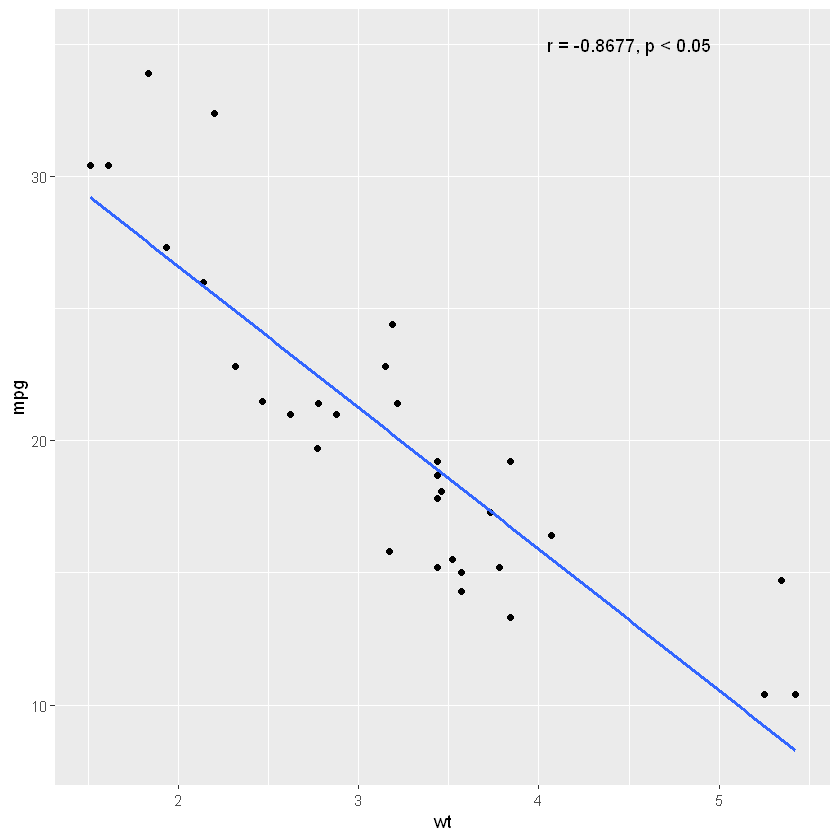#### More Complex Correlation Test and Text Example¶

In :
require(tidyr)
require(purrr)

data(Burt, package = 'carData')

corr <- Burt %>% group_by(class) %>%
nest() %>%
mutate(Cor = map(data, ~ cor.test(.$IQbio, .$IQfoster)),
p   = map_dbl(Cor, 'p.value'),
est = map_dbl(Cor, 'estimate')
) %>%
mutate_if(is.numeric, round, 4) %>%
select(class, p, est, Cor)

text <- corr %>%
mutate(
text = paste0('r = ', est, ', ',
ifelse(p <= 0.01,
'p < 0.05',
paste('p = ', p))))

Burt$class <- as.factor(Burt$class)

gp <- ggplot(aes(x = IQbio, y = IQfoster),
data = Burt)

corrp <- gp + geom_point(aes(color = class,
shape=class)) +
geom_smooth(aes(color = class), method = "lm", se = FALSE) +
geom_text(aes(x = 120, y = 137, color="high",
label=subset(text, class == "high")$text)) + geom_text(aes(x = 118, y = 109, color="medium", label=subset(text, class == "medium")$text)) +
geom_text(aes(x = 124, y = 103, color="low",
label=subset(text, class == "low")$text)) corrp  Loading required package: tidyr Loading required package: purrr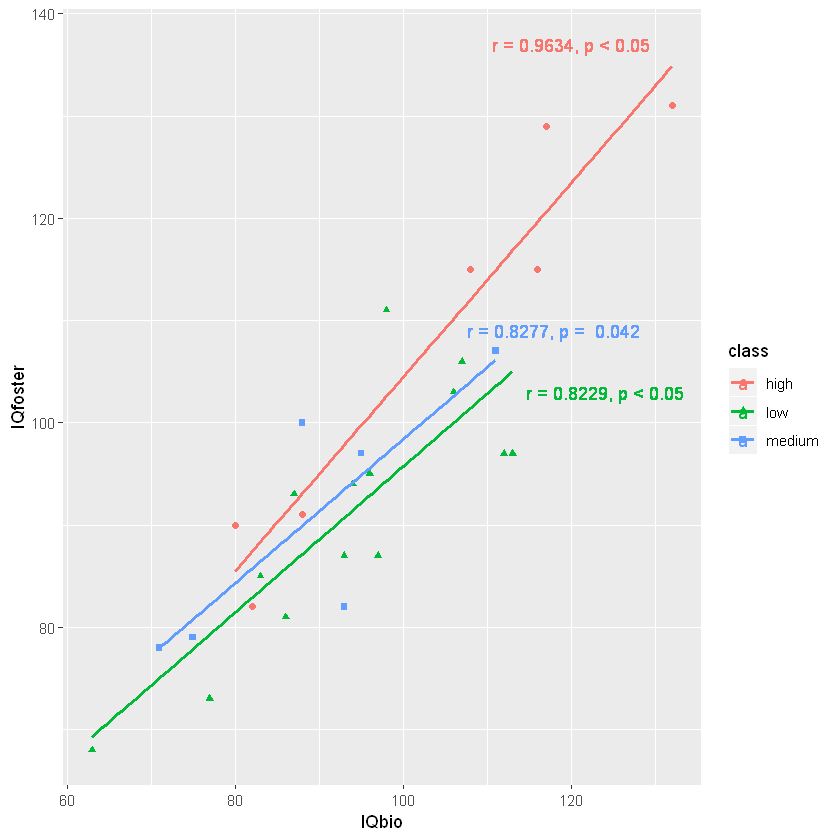### How to Rotate the Axis using Ggplot2¶ Here's how to rotate the axis labels In : data(Salaries, package = "carData") Salaries$rank <- as.factor(Salaries\$rank)

gp <- ggplot(aes(x = salary, y = yrs.since.phd),
data = Salaries) +
geom_point(aes(color = rank,
shape = rank)) +
geom_smooth(method = "lm") +
scale_y_continuous(limits = c(0, 60)) +
scale_x_continuous(limits = c(50000, 240000),
breaks = seq(50000, 240000, by = 10000))


To rotate the axis do this:

In :
gp + theme(axis.text.x =
element_text(angle = 90, hjust = 1))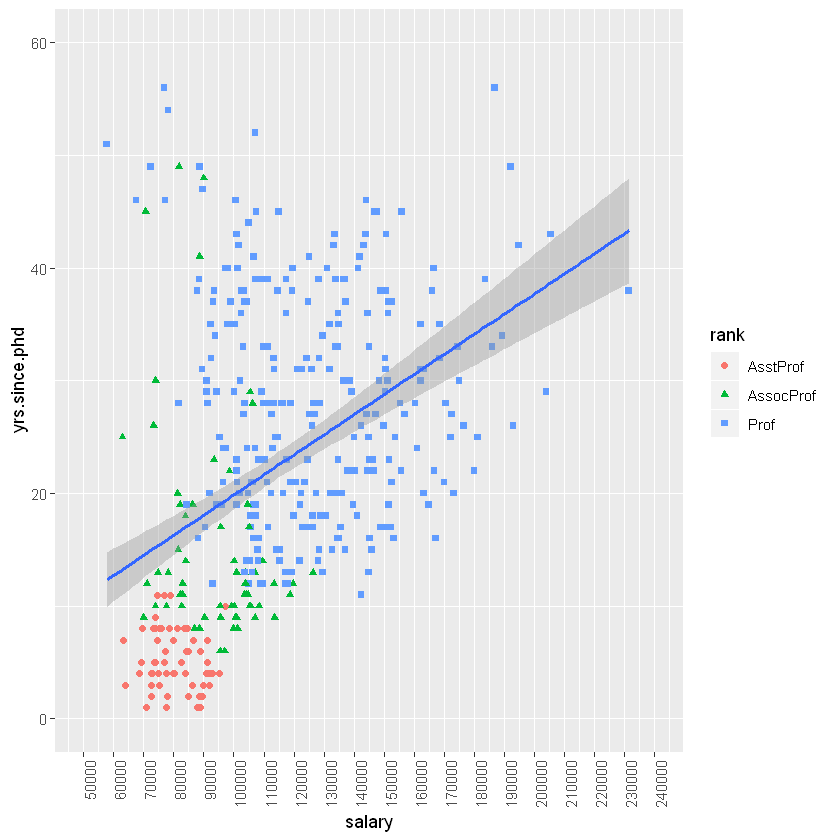### How to Style a Scatter plot in R¶

Here we use the theme_bw() function to get a dark-light themed plot. Then, we are going to make the scatter plot in black and grey colors using the scale_colour_grey() function. Finally, we add a theme layer using the function theme().

The function element_blank() will make draw “nothing” at that particular parameter. For instance, plot.background = element_blank() will give the plot a blank (white) background.

In :
corrp + theme_bw() +  scale_colour_grey() +
theme(axis.line = element_line(colour = "black")
,plot.background = element_blank()
,panel.grid.major = element_blank()
,panel.grid.minor = element_blank()
,strip.background = element_blank()
,panel.border = element_blank()
,legend.title=element_blank()
,legend.key = element_blank())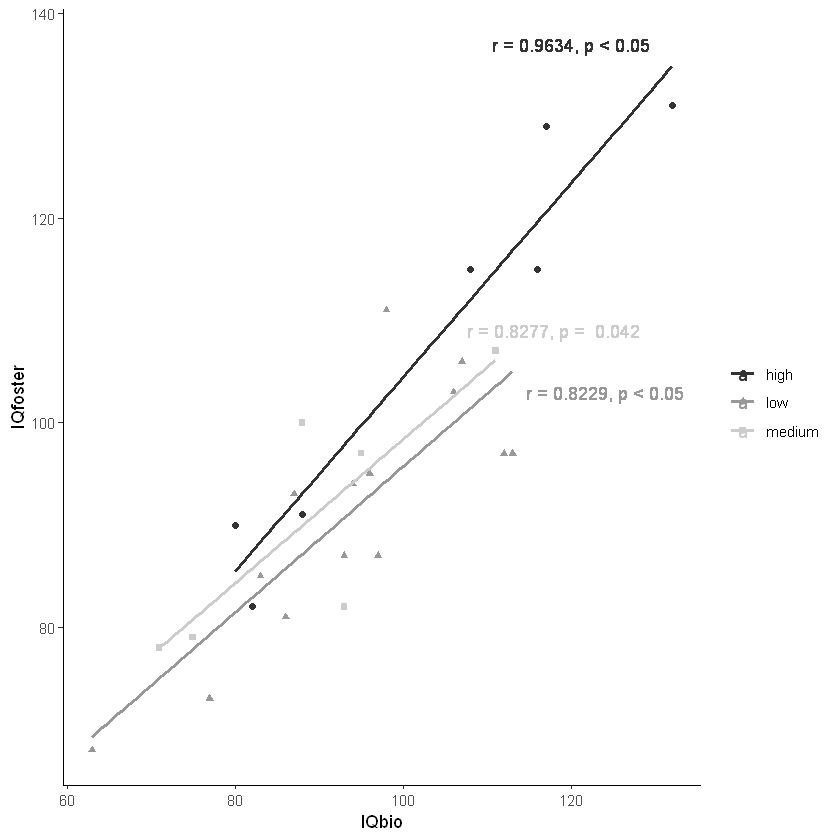#### Pairplot in R: Scatterplot + Histogram¶

Let's create the pairplots using the package GGally.

In :
require(GGally)

cols = c('mpg', 'wt', 'hp', 'qsec')
ggpairs(mtcars, columns = cols)

Loading required package: GGally
Warning message:
"package 'GGally' was built under R version 3.6.1"Registered S3 method overwritten by 'GGally':
method from
+.gg   ggplot2

Attaching package: 'GGally'

The following object is masked from 'package:dplyr':

nasa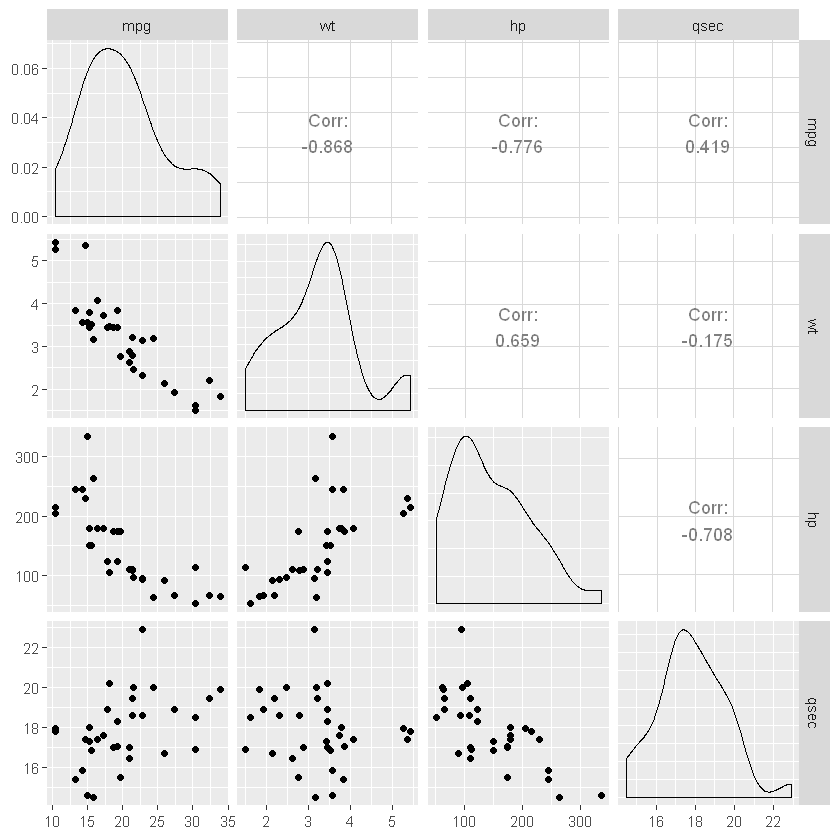### Saving a High Resolution Plot in R¶

In this section, we are going to learn how to save ggplot2 plots as PDF and TIFF files.

In :
data(Salaries, package = "carData")

gp <- ggplot(aes(x=yrs.since.phd, y=salary),
data=Salaries) +
geom_point() +
geom_smooth(method = "lm", se = FALSE, colour="gray") +
theme_bw() +
theme(axis.line = element_line(colour = "black")
,plot.background = element_blank()
,panel.grid.major = element_blank()
,panel.grid.minor = element_blank()
,strip.background = element_blank()
,panel.border = element_blank()
,legend.title=element_blank()
,legend.key = element_blank())  +
xlab('Years since Ph.D.') +
ylab('Salary')


Now we can use the ggsave() function to save the scatter plot.

### How to Save a Scatter Plot to PDF in R¶

Let's save a pdf!

In :
ggsave("salaries_by_year_scatterplot.pdf", device = "pdf",
width = 12, height = 8,
units = "cm", dpi = 300)


### How to Save a Scatter Plot to TIFF in R¶

Let's save a TIFF!

In :
ggsave("salaries_by_year_scatterplot.tiff", device = "tiff",
width = 12, height = 8,
units = "cm", dpi = 300)